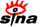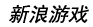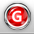下载新浪UT Game　客户端高速下载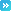推荐：全球新网游 | 网页游戏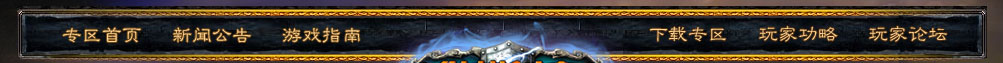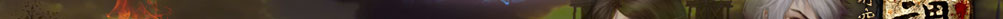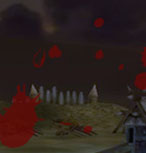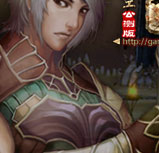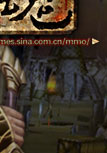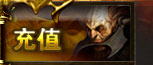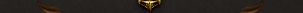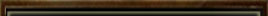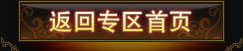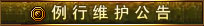为了保证游戏运行的稳定性及提升整体服务质量，《纵横时空》游戏全部服务器将每周二凌晨4：00-12：00进行例行停机维护， 停机期间给您带来的不便，敬请谅解。祝大家游戏愉快！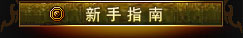登录游戏 创建角色 界面说明 基本操作 快 捷 键 角色属性 系统设置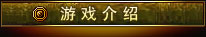背景故事 世界介绍 两大阵营 五大宗派 配置说明 下载安装 收费充值 Vista系统启动指南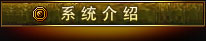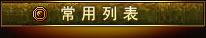游戏问题联系电话：            010-84799696 充值问题联系电话：客服电话：010-52926702/03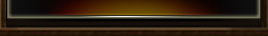# 祭牙平原

 任务名称 接受等级 给予任务NPC 阵营需求 任务类型 形势 >=10 巴青 正仪 主线任务 四处走走 >=10 巴青 正仪 主线任务 坠毁的飞船 >=10 巴青 正仪 主线任务 罗准将的忧虑 >=10 罗准将 正仪 主线任务 消灭斧头帮众 >=10 路中士 正仪 主线任务 回收物品 >=10 路中士 正仪 主线任务 传递便条 >=10 路中士 正仪 主线任务 罗准将的嘱托 >=10 罗准将 正仪 主线任务 谒见大书记官 >=11 巴青 正仪 主线任务 正仪与祭牙 >=11 曲静然 正仪 主线任务 发证 >=11 曲静然 正仪 主线任务 赴命 >=11 曲静然 正仪 主线任务 为民解忧 >=11 龙怖 正仪 主线任务 赶赴晶石谷 >=12 龙怖 正仪 主线任务 搜救行动 >=12 西全丰 正仪 主线任务 残缺的部署图I >=12 完烈 正仪 主线任务 残缺的部署图II >=13 西全丰 正仪 主线任务 进攻！进攻！ >=13 西全丰 正仪 主线任务 黑色图腾柱 >=13 西全丰 正仪 主线任务 递送军情信 >=14 西全丰 正仪 主线任务 木怪的蠢动 >=14 龙怖 正仪 主线任务 清除木灵 >=15 彦青 正仪 主线任务 夺回情报 >=15 彦青 正仪 主线任务 全面进攻 >=15 彦青 正仪 主线任务 递送军情信 >=15 彦青 正仪 主线任务 大书记官的召见 >=16 龙怖 正仪 主线任务 大统领的召见 >=16 曲静然 正仪 主线任务 检查通路 >=10 巴青 正仪 支线任务 被抢走的种子 >=10 柳娘 正仪 支线任务 讨厌的鸟 >=10 慕容禄 正仪 支线任务 击败白猿 >=10 巴青 正仪 支线任务 鸣蛇哨 >=10 小玉 正仪 支线任务 除蚁行动 >=10 巴青 正仪 支线任务 收集羽毛 >=11 针织女 正仪 支线任务 救死扶伤I >=10 陈智弟 正仪 支线任务 救死扶伤II >=10 王药师 正仪 支线任务 救死扶伤III >=10 王药师 正仪 支线任务 寻找魔草 >=12 王药师 正仪 支线任务 送药 >=12 王药师 正仪 支线任务 尚宏的委托 >=11 尚宏 正仪 支线任务 厨娘大显身手 >=11 尚宏 正仪 支线任务 寻找佐料 >=11 虫小京 正仪 支线任务 美味食物 >=11 虫小京 正仪 支线任务 赚钱的机会 >=11 展弓 正仪 支线任务 采集芍药 >=10 文宣 正仪 支线任务 缉拿要犯 >=10 龙怖 正仪 支线任务 迫不得已 >=10 守卫郑雄 正仪 支线任务 物归原主 >=10 守卫郑雄 正仪 支线任务 制作护身符 >=12 法塔 正仪 支线任务 世外高人 >=12 大壮 正仪 支线任务 一展身手 >=11 大壮 正仪 支线任务 价值连城 >=10 钱丰 正仪 支线任务 以毒还毒I >=10 曲静然 正仪 支线任务 以毒还毒II >=10 曲静然 正仪 支线任务 解放奴隶 >=10 曲静然 正仪 支线任务 走狗监工 >=10 龙怖 正仪 支线任务 寻找精铁 >=10 大壮 正仪 支线任务 反叛军的末日(jy) >=10 西全丰 正仪 支线任务 大家的烦恼 >=14 曲静然 正仪 支线任务 寻找纺线 >=14 针织女 正仪 支线任务 彩贝儿的委托 >=14 针织女 正仪 支线任务 美丽的晶石 >=12 彩贝儿 正仪 支线任务 铁匠的需求 >=14 彩贝儿 正仪 支线任务 锋利的匕首 >=14 大壮 正仪 支线任务 人民爱戴 >=14 大壮 正仪 支线任务 邪木妖的哀叫(jy) >=15 彦青 正仪 支线任务 救救我的儿子 >=10 英小逝 正仪 支线任务 慕容禄的疑惑 >=13 慕容禄 正仪 支线任务 玉佩的来源 >=13 彩贝儿 正仪 支线任务 消息的代价 >=13 展弓 正仪 支线任务 玉佩的秘密 >=12 展弓 正仪 支线任务 兄弟相认 >=13 林戈 正仪 支线任务 罗汉技能进阶III >=15 岩妹 正仪 支线任务 玄武技能进阶III >=15 痴刀 正仪 支线任务 祝由技能进阶III >=15 白雪丽 正仪 支线任务 采集技能 >=10 大壮 正仪 支线任务 采集技能 >=10 针织女 正仪 支线任务 采集技能 >=10 彩贝儿 正仪 支线任务 宗派传送员 >=10 曲静然 正仪 支线任务 宗派传送员 >=10 曲静然 正仪 支线任务 宗派传送员 >=10 曲静然 正仪 支线任务 开启传送点I >=10 祭牙营地传送员 正仪 支线任务 开启传送点II >=10 祭牙传送员 正仪 支线任务 技能任务 >=10 大壮 正仪 生活技能任务 技能任务 >=10 针织女 正仪 生活技能任务 技能任务 >=10 彩贝儿 正仪 生活技能任务 士兵职责 <=30+>=20 洛青剑 正仪 PVP阵营任务 斩首行动 >=40 洛青剑 正仪 PVP阵营任务 刺杀特使 <=40+>=30 洛青剑 正仪 PVP阵营任务 坐享其成 <=50+>=35 洛青剑 正仪 PVP阵营任务 勇士的试炼I >=20+<=40 龙怖 正仪 活动任务 勇士的荣耀 >=40+<=50 龙怖 正仪 活动任务 勇士的试炼II >=50+<=60 龙怖 正仪 活动任务 授勋 >=20 曲静然 正仪 PVP阵营任务 收粮 >=20 法塔 正仪 每日任务 文明碎片 >=20 杜中士 正仪 每日任务 行刺 >=20 龙怖 正仪 每日任务 宝藏的下落 >=30 洛青剑 正仪 每日任务 以牙还牙 >=30 洛青剑 正仪 每日任务 职责 <=30+>=20 洛青剑 正仪 每日任务 击杀将领 >=40 洛青剑 正仪 每日任务 刺杀 <=40+>=30 洛青剑 正仪 每日任务 拿来主义 <=50+>=35 洛青剑 正仪 每日任务 纵横大陆教育委员会 >=10 郝学文 正仪 每日任务 双倍大汇考 >=10 洛青剑 正仪 每日任务 时空沙场 >=21 曲静然 正仪 支线任务 兑换高级聚灵石 >=10 法塔 正仪 支线任务 兑换正仪战徽 >=10 法塔 正仪 支线任务 寻找兄弟 >=23+<=24 林戈 正仪 每日任务 传授经验 >=22+<=25 彦青 正仪 每日任务 未来人的建议 >=24+<=27 杜中士 正仪 每日任务 药品快递 >=26+<=28 王药师 正仪 每日任务 送信 >=33 士官张伟 正仪 阵营任务 军火运输事件 >=33 士官张伟 正仪 阵营任务 筹集战服 >=35+<=39 士官张伟 正仪 每日任务 怪异的图腾I >=37 士官张伟 正仪 阵营任务 怪异的图腾II >=37 士官张伟 正仪 阵营任务 密信 >=40 士官张伟 正仪 阵营任务 转达命令 >=40 士官张伟 正仪 阵营任务 委任书 >=42 中尉李冰 正仪 阵营任务 前去支援 >=44 中尉李冰 正仪 阵营任务 储备食物 >=44+<=50 中尉李冰 正仪 每日任务 间谍 >=46+<=50 中尉李冰 【返回首页】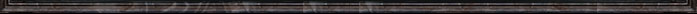留言 更多>>
 本新闻留言共条 显示 条
 登录名： 　密码： 　 匿名发表